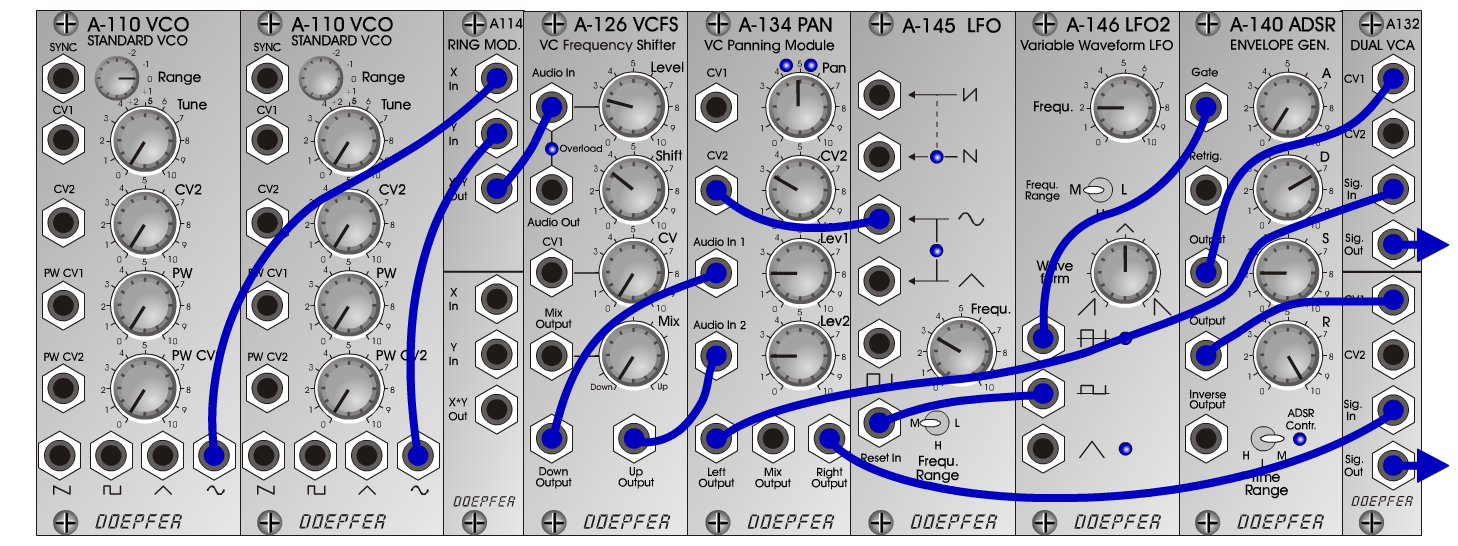Grandfather's ClockFXD o e p f e r A - 100 Connections: Settings: Grandfather's Clock 1: A-110/1 (Sine) <=> A-114 (X In)  A-110/2 (Sine) <=> A-114 (Y In)  A-114 (Out) <=> A-126 (Audio In)  A-126 (Down Output) <=> A-134 (Audio In 1)  A-126 (Up Output) <=> A-134 (Audio In 2)  A-134 (Right Output) <=> A-132/above (Sig. In)  A-134 (Left Output) <=> A-132/below (Sig. In)  A-132/top (Sig Out) <=> Amplifier right  A-132/bottom (Sig Out) <=> Amplifier left  A-146 (Pulse) <=> A-140 (Gate)  A-140 (Output A) <=> A-132/above (CV 1)  A-140 (Output B) <=> A-132/below (CV 1)  A-146 (positive-voltage Pulse) <=> A-145 (Reset In) A-110/1 (tune = 220 Hz)  A-110/2 (tune = 1318.5 Hz)  A-126 (Level = 2.5, Shift = 3.3)  A-145 (Frq = 3, Range = M)  A-140 (A = 0, D = 7, S = 2, R = 10, Range = M)  A-134 (Pan = 5, CV 2 = 3, Lev 1 = 2, Lev 2 = 2)  A-146 (Freq = 2, Wave Form = 5, Range = M) Grandfather's Clock 2: A-110/1 (tune = 220 Hz)  A-110/2 (tune = 659.25 Hz)  A-126 (Level = 2.5, Shift = 3.3) Grandfather's Clock 3: A-110/1 (tune = 220 Hz)  A-110/2 (tune = 659.25 Hz)  A-126 (Level = 2.5, Shift = 2.5) Grandfather's Clock 4: A-110/1 (tune = 220 Hz)  A-110/2 (tune = 659.25 Hz)  A-126 (Level = 2.5, Shift = 2.8) Grandfather's Clock 5: A-110/1 (tune = 220 Hz)  A-110/2 (tune = 659.25 Hz)  A-126 (Level = 2.5, Shift = 4.1) Grandfather's Clock 6: A-110/1 (tune = 220 Hz)  A-110/2 (tune = 659.25 Hz)  A-126 (Level = 2.5, Shift = 4.5) Grandfather's Clock 7: A-110/1 (tune = 220 Hz)  A-110/2 (tune = 659.25 Hz)  A-126 (Level = 2.5, Shift = 5.5) Grandfather's Clock 8: A-110/1 (tune = 110 Hz)  A-110/2 (tune = 329.63 Hz)  A-126 (Level = 2.5, Shift = 1.5) Grandfather's Clock 9: A-110/1 (tune = 110 Hz)  A-110/2 (tune = 329.63 Hz)  A-126 (Level = 2.5, Shift = 4.5) Grandfather's Clock 10: A-110/1 (tune = 110 Hz)  A-110/2 (tune = 329.63 Hz)  A-126 (Level = 2.5, Shift = 1.9) Grandfather's Clock 11: A-110/1 (tune = 110 Hz)  A-110/2 (tune = 329.63 Hz)  A-126 (Level = 2.5, Shift = 3.4) Josef MuellerSound samples Grandfather's Clock 1 Grandfather's Clock 2 Grandfather's Clock 3 Grandfather's Clock 4 Grandfather's Clock 5 Grandfather's Clock 6 Grandfather's Clock 7 Grandfather's Clock 8 Grandfather's Clock 9 Grandfather's Clock 10 Grandfather's Clock 11